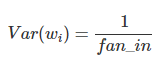# Xavier and He Normal (He-et-al) Initialization

Why shouldn’t you initialize the weights with zeroes or randomly (without knowing the distribution):

• If the weights in a network start too small, then the signal shrinks as it passes through each layer until it’s too tiny to be useful.
• If the weights in a network start too large, then the signal grows as it passes through each layer until it’s too massive to be useful.

Types of Initializations:

### Xavier/Glorot Initialization

Xavier Initialization initializes the weights in your network by drawing them from a distribution with zero mean and a specific variance,

where fan_in is the number of incoming neurons.

It draws samples from a truncated normal distribution centered on 0 with `stddev = sqrt(1 / fan_in)` where `fan_in` is the number of input units in the weight tensor.

Generally used with tanh activation.

Also generally,

is used where fan_out is the number of neurons the result is fed to.

### He Normal (He-et-al) Initialization

This method of initializing became famous through a paper submitted in 2015 by He-et-al, and is similar to Xavier initialization, with the factor multiplied by two. In this method, the weights are initialized keeping in mind the size of the previous layer which helps in attaining a global minimum of the cost function faster and more efficiently.The weights are still random but differ in range depending on the size of the previous layer of neurons. This provides a controlled initialization hence the faster and more efficient gradient descent.

if RELU activation:

It draws samples from a truncated normal distribution centered on 0 with `stddev = sqrt(2 / fan_in)` where `fan_in` is the number of input units in the weight tensor.

Proof why :

We have an input X with n components and a linear neuron with random weights Wand output Y.

can be found on Wikipedia

Now lets assume mean =0

since

and if we make a assumption of i.i.d., we get

So we want this Var(Y) =1

In Glorot & Bengio’s, If we go through the same steps for the backpropagated signal, we get

to keep the variance of the input gradient & the output gradient the same. These two constraints can only be satisfied simultaneously if fan_in=fan_out, so a compromise, we take the average of the two:

In a recent paper by He, Rang, Zhen and Sun they build on Glorot & Bengio and suggest using

### Implementations:

Numpy Initialization

`w=np.random.randn(layer_size[l],layer_size[l-1])*np.sqrt(1/layer_size[l-1])`
`w=np.random.randn(layer_size[l],layer_size[l-1])*np.sqrt(2/(layer_size[l-1]+layer_size[l]))`

Tensorflow Implementation

``tf.contrib.layers.xavier_initializer(    uniform=True,    seed=None,    dtype=tf.float32)``

This initializer is designed to keep the scale of the gradients roughly the same in all layers. In uniform distribution this ends up being the range: `x = sqrt(6. / (in + out)); [-x, x]` and for normal distribution a standard deviation of `sqrt(2. / (in + out))` is used.

You can use the below to use all types:

``tf.contrib.layers.variance_scaling_initializer(factor=2.0, mode='FAN_IN', uniform=False, seed=None, dtype=tf.float32)``
``if mode='FAN_IN': # Count only number of input connections.    n = fan_in  elif mode='FAN_OUT': # Count only number of output connections.    n = fan_out  elif mode='FAN_AVG': # Average number of inputs and output connections.    n = (fan_in + fan_out)/2.0    truncated_normal(shape, 0.0, stddev=sqrt(factor / n))``

Keras Initialization

• `tf.keras.initializers.glorot_normal(seed=None)`

It draws samples from a truncated normal distribution centered on 0 with `stddev = sqrt(2 / (fan_in + fan_out))`where `fan_in` is the number of input units in the weight tensor and `fan_out` is the number of output units in the weight tensor.

• `tf.keras.initializers.glorot_uniform(seed=None)`

It draws samples from a uniform distribution within [-limit, limit] where `limit` is `sqrt(6 / (fan_in + fan_out))`where `fan_in` is the number of input units in the weight tensor and `fan_out` is the number of output units in the weight tensor.

• `tf.keras.initializers.he_normal(seed=None)`

It draws samples from a truncated normal distribution centered on 0 with `stddev = sqrt(2 / fan_in)` where `fan_in`is the number of input units in the weight tensor.

• `tf.keras.initializers.he_uniform(seed=None)`

It draws samples from a uniform distribution within [-limit, limit] where `limit` is `sqrt(6 / fan_in)` where `fan_in` is the number of input units in the weight tensor.

• `tf.keras.initializers.lecun_normal(seed=None)`

It draws samples from a truncated normal distribution centered on 0 with `stddev = sqrt(1 / fan_in)` where `fan_in`is the number of input units in the weight tensor.

• `tf.keras.initializers.lecun_uniform(seed=None)`

It draws samples from a uniform distribution within [-limit, limit] where `limit` is `sqrt(3 / fan_in)` where `fan_in` is the number of input units in the weight tensor.

References:

Thrown in a like if you liked it to keep me motivated.

Source: Deep Learning on Medium